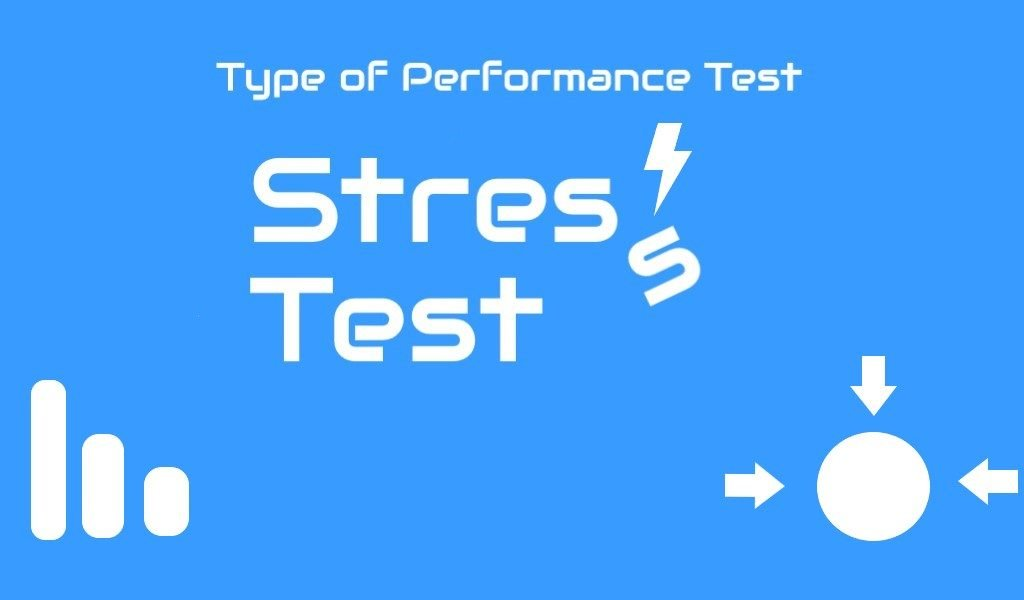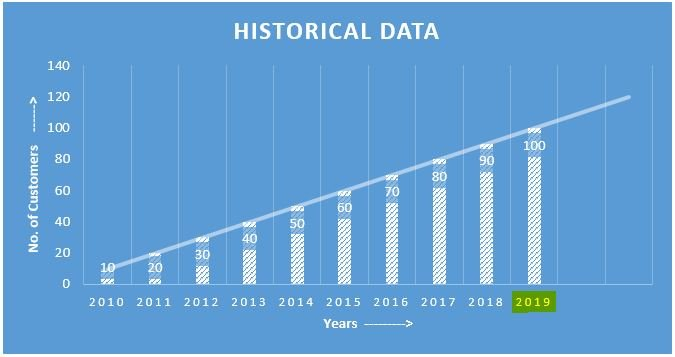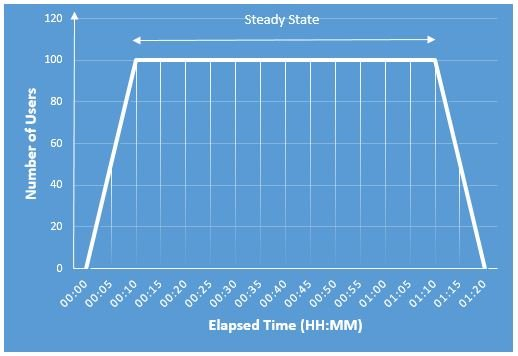# Stress Test## What is Stress Test?

Stress Test is a type of non-functional test which verifies the performance of an application or software system at futuristic load. The futuristic load is a predicted load which should be handled by an application in the future with existing software and hardware.  The defined load for a Stress test is always more than a Load Test.

### How to calculate the Future Load?

The future load is calculated with the help of historical data. The business analyst predicts the numbers by referring to previous years statistics. He calculates the figure by using some mathematical formulae. To understand the future load calculation refers to the linear graph shown below. It is a typical graph; not a practical one:Figure 1: A typical historical data for future load prediction (Linear Graph)

This graph shows the historical as well as predicted data. The historical data lies from the year of 2010 to 2018 and the year 2019 shows futuristic load. As per linear equation calculation, the predicted load is 100 for 2019. Hence 100 is the user load for the stress test.

In some cases, if data is not available for the calculation then 125%, 150% or 200% of peak load can be considered for the stress test. But before conducting the test get the confirmation on these volumes from the project team.

For a new application: Since a new application does not have any historical data, so the load test fulfils the purpose of the stress test. Because a new application has predicted peak load which is as same as the future load. If the project has a long term goal for a new application and wants to test it beyond the predicted peak load then the successive tests of load test will be considered as stress tests. These successive test should have a higher user volume than load test.

## Purpose of Stress Test:

1. To Identify whether the application can handle the future load
2. Note the response time at future load
3. To check whether the behaviour of resources (CPU, Memory and Disk). They should not breach the defined performance limit
4. To identify if there is any bottleneck
5. Note down the error percentage

## Approach:

NFR document has a separate set of NFRs for the stress test. These NFRs are related to the count of future user load, response time, transactions per second etc. In the absence of future load, conduct the test at 125%, 150% or 200% percentage of peak load and analyse the behaviour of the application.  A performance tester designs the workload model using defined or calculated NFRs and executes the test. Ideally, the duration of the stress test is 1 hour (excluding ramp-up and ramp-down period). A typical stress test user graph is:Figure 2: A Typical Stress Test Graph

This stress test graph has a steady state of 1 hour along with 10 minutes ramp-up and 10 minutes ramp-down period. Therefore the test will run for 1 hour and 20 minutes. After completion of the test, a performance tester verifies the result against the defined load test NFRs.

It is recommended to plan at least 2 stress tests in a single performance testing cycle and if both the tests have consistent results then only jump on to next type of performance test.

### 2 Responses

1.Ravina Kalyanshetty says:

In the absence of future load, conduct the test at 125%, 150% or 200% percentage of peak load and analyse the behaviour of the application.
Can you elaborate more on this above statement.

•PerfMatrix says:

Hi Ravina,

In case peak load is given 100, then you can run stress tests with 125, 150 or 200 user load which are 125%, 150% and 200% of the peak load.